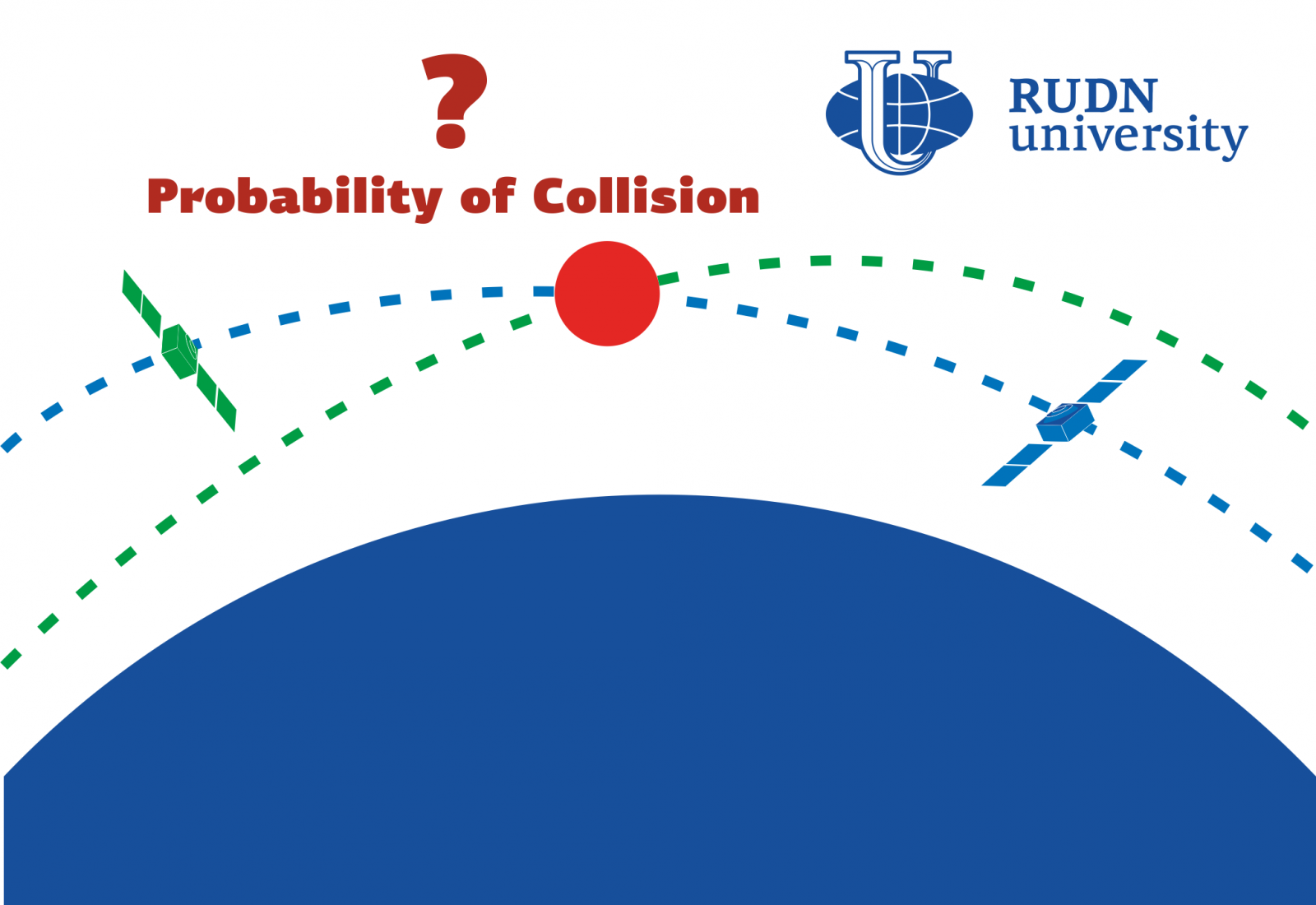RUDN scientists have shown how much taking into account the real shape of the satellite affects the probability of collision of two space objects

# RUDN scientists have shown how much taking into account the real shape of the satellite affects the probability of collision of two space objects

RUDN scientists have clarified the model for calculating the probability that space objects may encounter. Classical models do not take into account the real shape and orientation of objects -it seemed that this increases the calculated probability several times. The results are published in the journal Cosmic Research.

Every year, space mission control centers register up to 70 potentially dangerous spacecraft rendezvous. To avoid collisions, they create specializedsystems, but they calculate the probability of collision with some assumptions. For example, objects are considered to have spherical shapes,their velocities are determined without errors, and so on. These assumptions reduce the accuracy of calculations.

“The assumptions of these approaches can significantly affect the magnitude of the probability of collision. For example, the assumption that space objects move in a straight line within the rendezvous interval does not allow us to estimate convergences, the duration of which takesa long time. Other assumptions also make a significant contribution to the error of calculations. You can remove these limitations by resorting to statistical modeling methods, ”-Maxim Karatunov, Candidate of Technical Sciences, Associate Professor of the Department of Mechanics and Mechatronics, RUDN University.

RUDN scientists considered a model that takes into account the real shape and orientation of space objects. The probability of collision was found using static modeling using the Monte Carlo method. Its essence lies in the use of a random variable generator and multiple calculation of themodel, and the results obtained determine the probabilityof theprocess, in this case — the collision. Scientists used the model to calculate the probability of collision of two real objects, Chinese geostationary satellites CHINASAT-20 and SHIJIAN-17.

The chinaSAT-20 and SHIJIAN-17 satellites approached on March 16, 2018 by 286.3 meters. To test the algorithm, the scientists first calculated the probability of a collision without taking into account the real form (believing that both satellites look like spheres), and then repeated the calculations with a more realistically approximate form. Scientists used a generalized model of the satellite — the body and “wings” of solar panels. Batteries for simplicity were presented in the form of cylinders, given that they have only one degree of freedom (that is, they can rotate around the axis of this cylinder). The researchers also took into account the orientation of the object — the main axis is directed to the Earth, and the axes of the cylinder-batteries are perpendicular to the plane of the orbit. The probability of a collision was five times less than in the classical calculation.

“The probability of collision of space objects is the main value that affects the decision to maneuver. The safety of space flights depends on the accuracy of the assessment of this value. We have developed a method for calculating probability, which allows usto take into account the shape and orientation of converging objects, as well as remove a number of classical assumptions. It was demonstrated that ignoring the real shape of objects significantly affects the accuracy of the assessment, for the test example, a five-fold discrepancy in the results was obtained, ”- maximKaratunov, Associate Professor of the Department of Mechanics and MEchatronics rudn University.

The results are published in the journal Cosmic Research.News
All news
Science
02 May
The Grand Prix and gold of the Hi-Tech exhibition go to RUDN dentists

April 18-20, the international exhibition of HI-TECH innovations took place in St. Petersburg. Two RUDN University projects received a gold medal and the only Grand Prix at the exhibition.

Science
14 Apr
RUDN Biologists Study Live Microorganisms in Toxic Liquids for Metalworking

RUDN biologists have studied microorganisms that can survive in metalworking fluids. The results will allow “picking up” bacteria and fungi that can process toxic waste fluids into a harmless product.

Science
10 Apr
RUDN University Biologist: salted water saves fish from stress when transporting

RUDN University biologist with colleagues from Brazil and Iran found how to alleviate the stress of fish during transportation. It turned out that this can be achieved with salted water.